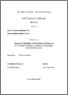# Numerical Modelling of Point-Masses Sliding on 2D Complex Surfaces as a Means to Investigate Rockslide Dynamics

Elsen, Katharina Maria (2017) Numerical Modelling of Point-Masses Sliding on 2D Complex Surfaces as a Means to Investigate Rockslide Dynamics, [Dissertation thesis], Alma Mater Studiorum Università di Bologna. Dottorato di ricerca in Geofisica, 28 Ciclo. DOI 10.6092/unibo/amsdottorato/8133.
Documenti full-text disponibili:Anteprima
Documento PDF (English) - Richiede un lettore di PDF come Xpdf o Adobe Acrobat Reader
Disponibile con Licenza: Creative Commons Attribution Non-commercial Share Alike 3.0 (CC BY-NC-SA 3.0) .

## Abstract

The present work introduces a new Lagrangian method to solve the equations of motion which model gravitational sliding of point masses on piecewise linear curves and piecewise planar surfaces. This method shall be incorporated in the already existing UBO-block model which can be used to model rockslide dynamics. In the first case, the equations are solved for a curve, which is approximated by means of a continuous piecewise defined curve \$\Sigma\$ formed by straight segments. In this special case, the solution can be computed analytically, dividing it into parts, one being the motion along the single segments and another being the effect of the transition between two segments. This is possible as the equations consist of two types of terms: those containing gravity acceleration \$g\$ but not containing derivatives of second order, and those that do contain second order derivatives, but not \$g\$. While the first terms determine the motion along the piecewise linear/planar segments, the second ones are sufficient to describe the transition between the elements. In a second step, the method was generalized to the 2-dimensional case for which a semi-analytical solution to the equations was derived. While the transition between two planar elements can be computed exactly, the motion along planar segments generally must be computed numerically. The method was implemented in a modular way in modern Fortran making use of typical HPC optimization methods. The goodness of the method is discussed by comparing the solutions to the ones computed for smooth curves and surfaces using the LSODE-solver for the time-integration. It is concluded that the new method significantly reduces the computational costs while reaching fully comparable results. The dependency of the solution on friction, grid resolution and initial position as well as its sensitivity to grid regularity were investigated using Monte-Carlo simulations.

Abstract
Tipologia del documento
Tesi di dottorato
Autore
Elsen, Katharina Maria
Supervisore
Dottorato di ricerca
Scuola di dottorato
Scienze matematiche, fisiche ed astronomiche
Ciclo
28
Coordinatore
Settore disciplinare
Settore concorsuale
Parole chiave
gravitational sliding, point mass, numerical modelling, landslides
URN:NBN
DOI
10.6092/unibo/amsdottorato/8133
Data di discussione
23 Maggio 2017
URI